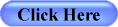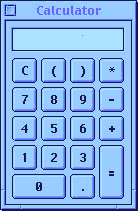# Health Tests and Calculators

 Food Item Calorie CalculatorCoronary Heart Disease Risk CalculatorEye Tests (Early detection is the key to minimizing vision loss)Cholesterol Risk CalculatorBody, Nutrition and Fitness CalculatorTry short ( approximately 2 minutes) free computerized BRAIN AGE vs. BRAIN POWER test now
try Life Expectancy Calculator now

Different types of calculators you may need:
• BMI Calculator (Body Mass Index calculator )
• Calorie Calculator
• DAS (Disease Activity Score) Calculator
• CrCL (Creatine Clearance Calculator)
• GFR (Glomerular Filtration Rate) Calculator
• Health risk assessment calculator
• Arthritis risk calculator
• Waist to Hip Ratio calculator
• Calories Burned Today calculator
• Calorie Adjustment calculator
• Ideal Weight calculator
• Gain Muscle Weight calculator
• Heart Attack Risk calculator
• Blood Alcohol Calculator
• Body Fat Estimator
• Optimal weight calculator